﻿﻿ دسترسی همگانی(OPAC) نام کتابخانه در اوپک

### رکوردهای مرتبط با این فروست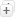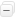مرتب سازی     درج پیشنهاد خرید   پالایش جستجو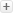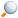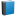Schaum's outline of theory and problems of heat transfer (1983) / PITTS ، DONALD R، نویسندهSchaum's Outline of theory and problems of programming with BASIC (1986) / Gottfried ، Byron S (1934)، نویسندهSchaum's outline of theory and problems of engineering mechanics (1962) / McLean ، W. G. (William G.)، نویسندهSchaum's outline of theory and problems of feedback and control systems (1967) / DiStefano ، Joseph J، نویسندهMathematical handbook of formulas and tables (1968) / Spiegel ، Murray R، نویسنده